Actions

An integral transform of a function in several variables, related to the Fourier transform. It was introduced by J. Radon (see ).

Let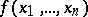be a continuous function of the real variables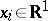that is decreasing sufficiently rapidly at infinity,,.

For any hyperplane in,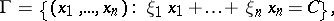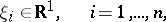andthe following integral is defined: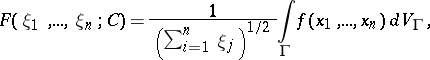whereis the Euclidean-dimensional volume in the hyperplane. The functionis called the Radon transform of the function. It is a homogeneous function of its variables of degree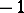: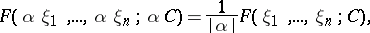and is related to the Fourier transform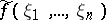,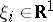, ofby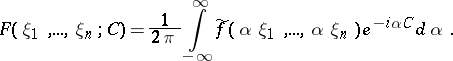The Radon transform is immediately associated with the problem, going back to Radon, of the recovery of a functionfrom the values of its integrals calculated over all hyperplanes of the space(that is, the problem of the inversion of the Radon transform).